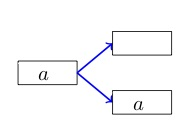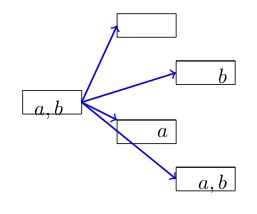Subsets Proper and Improper

Question

How many subsets can be formed from a set of four elements, say ? How many proper subsets?

Solution

Terminology

An improper subset includes all the elements in the original set. In other words, an improper subset of set is set itself.

A proper subset of set can be any subset of set except the improper subset.

If “proper” is not specified, “subset” is understood to include the improper subset.

Approach

Consider a set with just one element: Set . How many subsets does it have? Remember that the empty set is a subset of every set. So set  has two subsets:  (the empty set) and . The set that includes  is improper, and is proper.Now think about a set with two elements: Set . ‘s subsets include both of ’s subsets,  and . And ’s subsets also include each of ’s subsets with element  added to it – resulting in subsets  and  – for a total of four subsets, including three proper subsets.Does the pattern continue? Set . ’s subsets include all four of ’s subsets, {}, {a}, {b}, and {a, b}; as well as each of ’s subsets with element c added to it – {c}, {a, c}, {b, c}, and {a, b, c} – for a total of eight subsets, including seven proper subsets.Each time an element is added to the set, the number of subsets doubles. If there’s just one element, there are two subsets; for two elements, there are subsets; for three elements, there are subsets, and for n elements, there are subsets.

Each set has one improper subset — that is, just one subset that contains all of the original set’s elements — so the number of proper subsets in each set of n elements is the number of subsets minus one, or .

Formulas

The number, , of subsets (including the improper subset) that can be formed from a set of n members is .

The number of proper subsets that can be formed from a set of n members is .

How many subsets can be formed of a set of four elements? .

How many proper subsets? .

16 subsets, including 15 proper subsets.